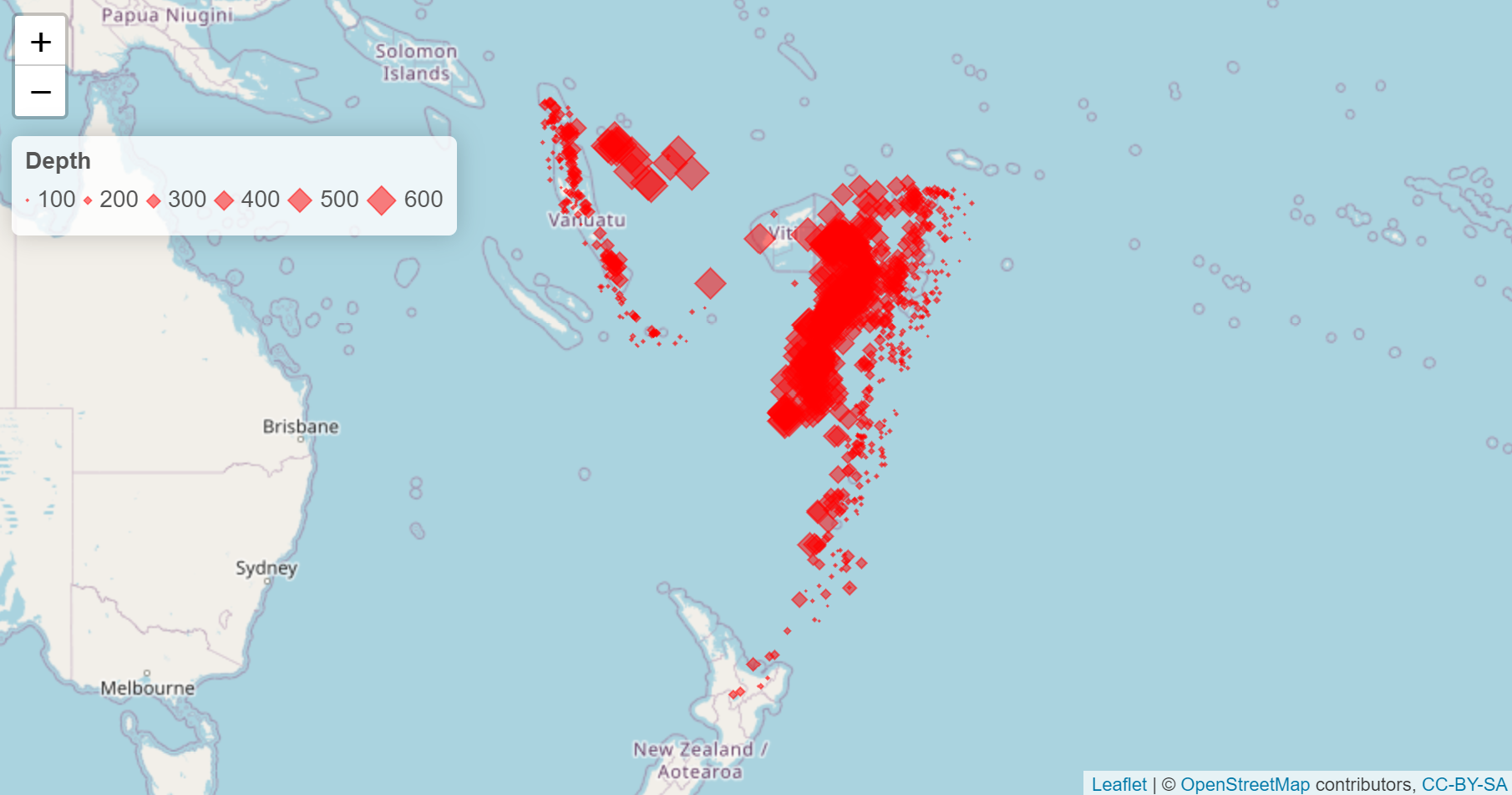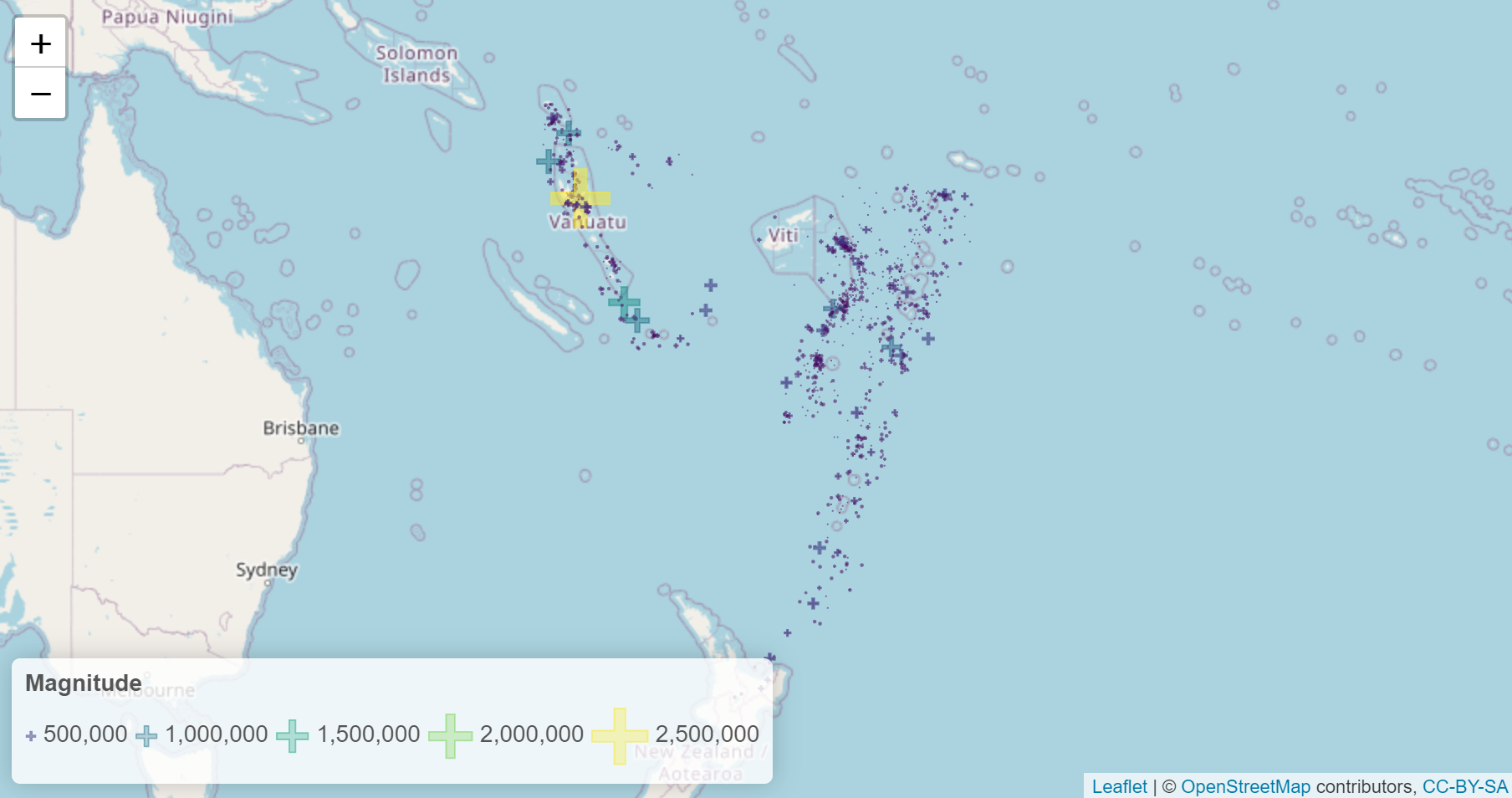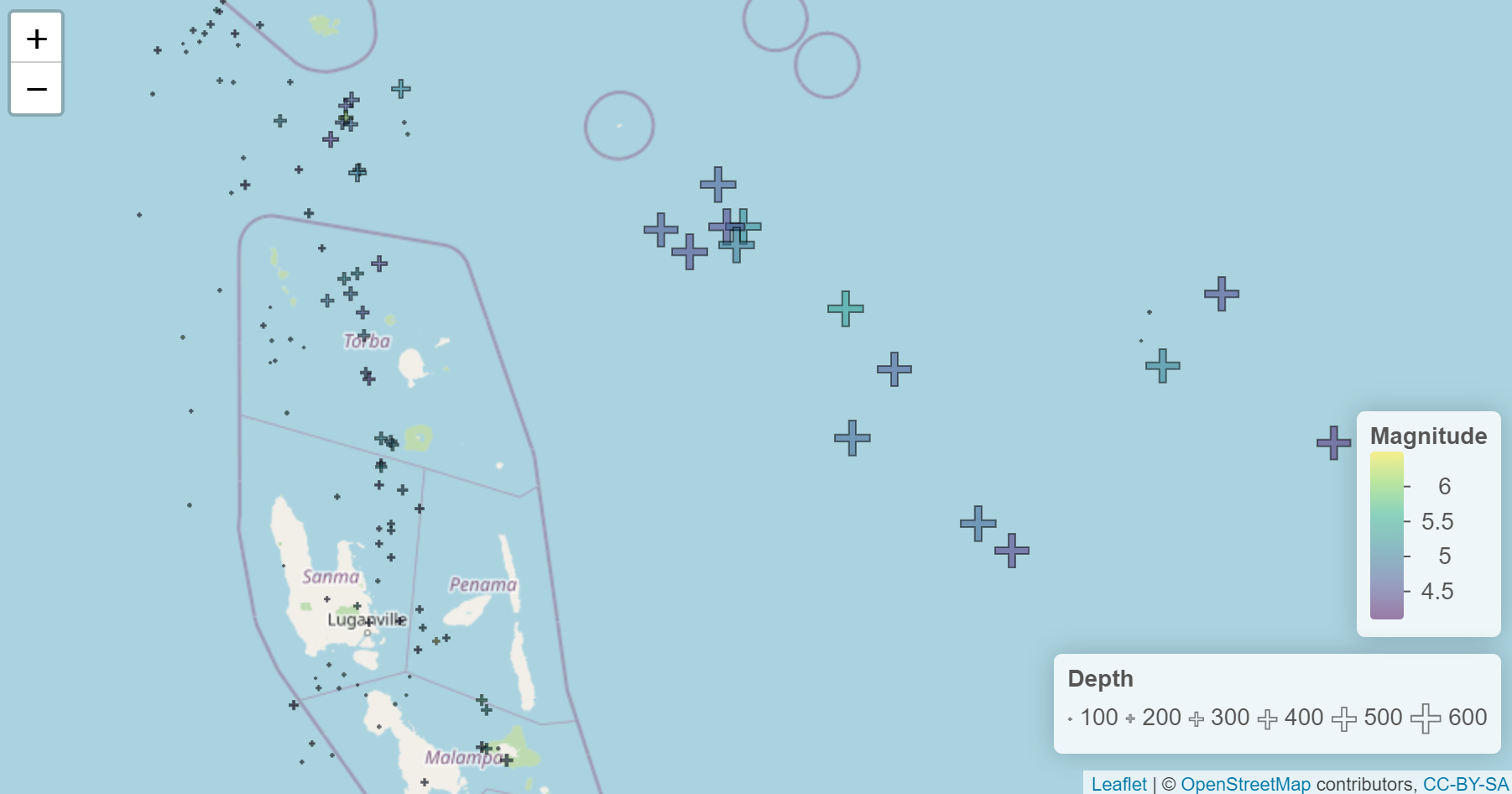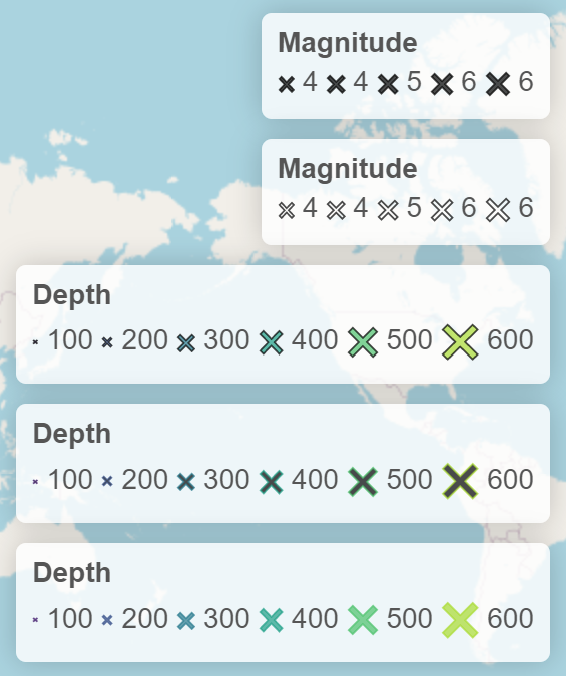Requires leaflegend >= 1.0.0

The leaflet package in R has built-in functionality for creating color encoded geometries and annotating with color legends. Support is lacking in the area of providing the ability to encode data with sizes or symbology except for the case of circle markers. Size and symbology are an important part of data visualization on a map. Using the leaflegend package, you can add both to your leaflet maps without adding external css or javascript.

# Adding Proportional Sizing to Symbols

To create the appropriately sized symbols, The makeSymbolsSize function can create a list of icons based on the output of sizeNumeric. The baseSize argument sets the center point size of the data scale to the mean of the values e.g. with values 1, 2, 3, 4, 5 and a base size of 10, the resultant size in pixels of the symbols are 3.33, 6.67, 10, 13.33, 16.67. The symbols can be added as icons to the map since they are svg elements. Some transparency is added here to show overlapping symbols.

To add a legend for the sizes, addLegendSize takes in the same values and uses the pretty function and breaks argument to create the scale. There may be some inconsistency between the number of breaks specified (leaflet internals also make use of the pretty function). It is easy to customize other aspects of the legend for appearance such as here: the color, symbol, and transparency are matched to the symbols and we align the legend items horizontally.

library(leaflet)
library(leaflegend)
data("quakes")
symbols <- makeSymbolsSize(
values = quakes$depth, shape = 'diamond', color = 'red', fillColor = 'red', opacity = .5, baseSize = 10 ) leaflet() %>% addTiles() %>% addMarkers(data = quakes, icon = symbols, lat = ~lat, lng = ~long) %>% addLegendSize( values = quakes$depth,
color = 'red',
fillColor = 'red',
opacity = .5,
title = 'Depth',
shape = 'diamond',
orientation = 'horizontal',
breaks = 5)# Encoding Color and Size

A helper function addSymbolsSize simplifies the above code and one can use a palette to generate colors. The symbols are switched to a plus sign to provide a smaller impact of area for overlapping points. The result is called double-encoding and is a way to emphasize differences in data. Because the Richter scale is on a logarithmic scale, the actual reading of magnitude was taken as ten to the power of.

numPal <- colorNumeric('viridis', 10^(quakes$mag)) leaflet(quakes) %>% addTiles() %>% addSymbolsSize(values = ~10^(mag), lat = ~lat, lng = ~long, shape = 'plus', color = ~numPal(10^(mag)), fillColor = ~numPal(10^(mag)), opacity = .5, baseSize = 1) %>% addLegendSize( values = ~10^(mag), pal = numPal, title = 'Magnitude', baseSize = 1, shape = 'plus', orientation = 'horizontal', opacity = .5, fillOpacity = .3, position = 'bottomleft', breaks = 5)# Split Color and Size Encodings It is also possible to color encode and size encode with different values. Using a palette function from the leaflet package, the color/fillColor arguments can be used to base the color encoding on a different vector of data than the values argument which is for size. numPal <- colorNumeric('viridis', quakes$mag)
leaflet(quakes) %>%
lat = ~lat,
lng = ~long,
shape = 'plus',
color = 'black',
fillColor = ~numPal(mag),
opacity = .5,
baseSize = 10) %>%
values = quakes$depth, color = 'black', title = 'Depth', shape = 'plus', orientation = 'horizontal', opacity = .5, fillOpacity = 0, breaks = 5, position = 'bottomright') %>% addLegendNumeric( pal = numPal, title = 'Magnitude', shape = 'stadium', values = quakes$mag,
fillOpacity = .5,
decreasing = TRUE,
position = 'bottomright')# Size Legend Options

Specifying color and fillColor will override any palette function that is provided. Below are examples of all the different configurations for the legend. The symbols are also available for makeSizeIcons to match the desired legend.

numPal <- colorNumeric('viridis', quakes$depth) leaflet() %>% addTiles() %>% addLegendSize( values = quakes$mag,
color = 'black',
title = 'Magnitude',
labelStyle = 'margin: auto;',
shape = 'cross',
orientation = 'horizontal',
opacity = .7,
numberFormat = function(x) {
prettyNum(x, big.mark = ",", scientific = FALSE, digits = 3)},
breaks = 5,
position = 'topright') %>%
values = quakes$mag, color = 'black', fillColor = 'transparent', title = 'Magnitude', labelStyle = 'margin: auto;', shape = 'cross', orientation = 'horizontal', opacity = .7, numberFormat = function(x) { prettyNum(x, big.mark = ",", scientific = FALSE, digits = 3)}, breaks = 5, position = 'topright') %>% addLegendSize( values = quakes$depth,
pal = numPal,
title = 'Depth',
labelStyle = 'margin: auto;',
shape = c('cross'),
orientation ='horizontal',
opacity = .7,
color = 'black',
breaks = 5,
position = 'topright') %>%
values = quakes$depth, pal = numPal, title = 'Depth', labelStyle = 'margin: auto;', shape = c('cross'), orientation ='horizontal', opacity = .7, fillColor = 'black', breaks = 5, position = 'topright') %>% addLegendSize( values = quakes$depth,
pal = numPal,
title = 'Depth',
labelStyle = 'margin: auto;',
shape = c('cross'),
orientation ='horizontal',
opacity = .7,
breaks = 5,
position = 'topright')[CRAN] [github]